# Shift dynamical system

(diff) ← Older revision | Latest revision (diff) | Newer revision → (diff)

A dynamical system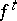(or, in a different notation,) on a space of continuous functions(is a metric space) equipped with the compact-open topology (that is, the topology of uniform convergence on segments), defined by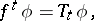whereis the shift operator by, that is,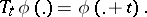Thus, the trajectory of a pointin a shift dynamical system is the set of all shifts of, that is, of all functions of the formfor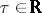. The closure of the trajectory is the set of all functions of the form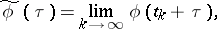where the limit is uniform on each segment. A shift dynamical system is equipped with normalized invariant measures (cf. Invariant measure); these exist by the Bogolyubov–Krylov theorem (Bogolyubov–Krylov invariant measures are concentrated on compact sets).

A shift dynamical system is used in the theory of dynamical systems mainly to construct examples (hereis usually taken to be; Markov's example of a non-strictly ergodic system on a compact set each trajectory of which is everywhere dense, and others), and also in the theory of non-autonomous systems of ordinary differential equations, whereis usually taken to beor a space of mappings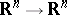(in the theory of linear homogeneous non-autonomous systems it is usual to take).

A shift dynamical system as defined above is often called a Bebutov system; cf. [a3]. The Bebutov–Kakutani theorem states that a dynamical system on a compact metric space is isomorphic to a subsystem of the Bebutov system withif and only if the set of its invariant points is homeomorphic to a subset of(cf. [a5], and, for a generalization, [a4]).
Usually, by a shift dynamical system one understands a discrete-time system (a cascade) of the form; heredenotes a finite non-empty set,is the space of all two-sided infinite sequences with elements in, endowed with the usual product topology (this is just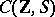with its compact-open topology whenis considered with its discrete topology), andis the shift operator by 1, that is,for.
These (discrete) shift systems play an important role in ergodic theory and topological dynamics. For example, a Bernoulli system is a shift system endowed with the product measure ondefined by a probability measure on(cf. Bernoulli automorphism). The discrete shift systems and their subsystems (subshifts) are not only used for the construction of special examples (for an important method — substitution — cf. [a6]), they are also important for the study of the behaviour of a large class of cascades by "coding" their trajectories by means of elements of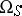for a suitable set(cf. Symbolic dynamics). More recently, it turned out that the methods used for the classification of so-called subshifts of finite type (see [a2]) are useful for information processing; see [a1].